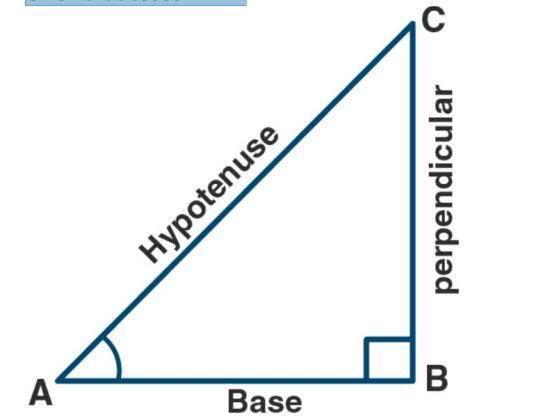# ML Aggarwal Solutions Class 9 Mathematics Solutions for Trigonometric Ratios Exercise 17 in Chapter 17 - Trigonometric Ratios

Question 12 Trigonometric Ratios Exercise 17

Given A is an acute angle and cosec A = √2, find the value of

2 \sin ^{2} A+3 \cot ^{2} A /

\left(\tan ^{2} A-\cos ^{2} A\right)

The values of all trigonometric functions dependent on the value of the ratio of sides in a right-angled triangle are known as trigonometric ratios. The trigonometric ratios of a right-angled triangle's sides with regard to any of its acute angles are known as that angle's trigonometric ratios.

Let triangle ABC be a right-angled at B and A is an acute angle.

Given that cosec A = √2Which implies,

AC/BC = √2/1

Let AC = √2x

Then BC = x

In right angled triangle ABC

By using Pythagoras theorem,

We get

\begin{array}{l} A C^{2}=A B^{2}+B C^{2} \\ (\sqrt{2} x)^{2}=A B^{2}+x^{2} \\ A B^{2}=2 x^{2}-x^{2} \\ A B=x \end{array}

Sin A = perpendicular/ hypotenuse

= BC/AC

= 1/ √2

Cot A = base/ perpendicular

= x/x

= 1

Tan A = perpendicular/ base

= BC/AB

= x/x

= 1

Cos A = base/ hypotenuse

= AB/AC

= x/ √2x

= 1/√2

Substituting these values we get

2 \sin ^{2} A+3 \cot ^{2} A /\left(\tan ^{2} A-\cos ^{2} A\right)=8

Related Questions
Exercises

Lido

Courses

Teachers

Book a Demo with us

Syllabus

Maths
CBSE
Maths
ICSE
Science
CBSE

Science
ICSE
English
CBSE
English
ICSE
Coding

Terms & Policies

Selina Question Bank

Maths
Physics
Biology

Allied Question Bank

Chemistry
Connect with us on social media!